June 8, 2022

# Tutorial: Plotting Data with Pandas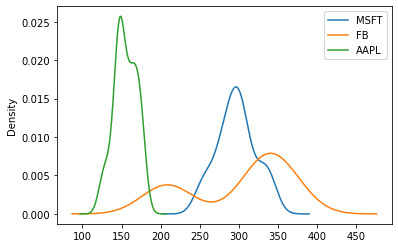## Pandas is a data analysis tool that also offers great options for data visualization. Here’s how to get started plotting in Pandas.

Data visualization is an essential step in making data science projects successful — an effective plot tells a thousand words.

Data visualization is a powerful way to capture trends and share the insights gained from data. There are plenty of data visualization tools on the shelf with a lot of outstanding features, but in this tutorial, we’re going to learn plotting with the Pandas package.

As you may already know, Pandas is a data analysis tool, but it provides some great options for data visualization. At the end of this tutorial, you’ll see how easy and straightforward plotting with Pandas can be.

We assume that you know the fundamentals of Pandas DataFrames. If you’re not familiar with the Pandas library, you might like to try our Pandas and NumPy Fundamentals course.

Let’s dive in.

## The Pandas `plot()` Method

Pandas comes with a couple of plotting functionalities applicable on DataFrame- or series objects that use the Matplotlib library under the hood, which means any plot created by the Pandas library is a Matplotlib object.

Technically, the Pandas `plot()` method provides a set of plot styles through the `kind` keyword argument to create decent-looking plots. The default value of the `kind` argument is the `line` string value. However, there are eleven different string values that can be assigned to the `kind` argument, which determines what kind of plot we’ll create.

The `.plot` is also an attribute of Pandas DataFrame and series objects, providing a small subset of plots available with Matplotlib. In fact, Pandas makes plotting as simple as just writing a single line of code by automating much of the data visualization procedure for us.

## Importing the Libraries and the Dataset

In this tutorial, we’re going to work on the weekly closing price of the Facebook, Microsoft, and Apple stocks over the last previous months. The following code imports the necessary libraries and the dataset required for visualization and then displays the content of the DataFrame on the output. The `%matplotlib inline` magic command is also added to the code to ensure the plotted figures appear in the notebook cells correctly:

``````import pandas as pd
import numpy as np
import matplotlib.pyplot as plt

dataset_url = ('https://raw.githubusercontent.com/m-mehdi/pandas_tutorials/main/weekly_stocks.csv')
pd.set_option('display.max.columns', None)
``````                  MSFT          FB        AAPL
Date
2021-05-24  249.679993  328.730011  124.610001
2021-05-31  250.789993  330.350006  125.889999
2021-06-07  257.890015  331.260010  127.349998
2021-06-14  259.429993  329.660004  130.460007
2021-06-21  265.019989  341.369995  133.110001``````

We’re now ready to explore and visualize the data with Pandas.

### Line Plot

The default plot is the line plot that plots the index on the x-axis and the other numeric columns in the DataFrame on the y-axis.

Let’s plot a line plot and see how Microsoft performed over the previous 12 months:

``df.plot(y='MSFT', figsize=(9,6))``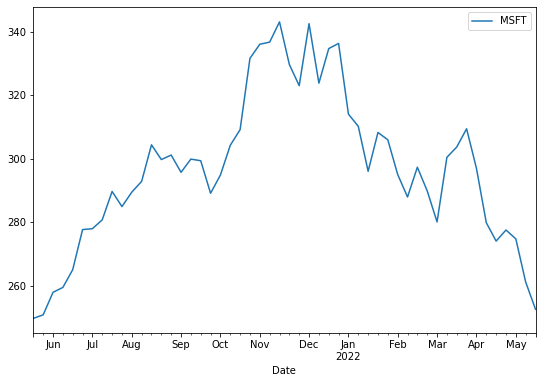NOTE

The `figsize` argument takes two arguments, width and height in inches, and allows us to change the size of the output figure. The default values of the width and height are 6.4 and 4.8, respectively.

We can plot multiple lines from the data by providing a list of column names and assigning it to the y-axis. For example, let’s see how the three companies performed over the previous year:

``df.plot.line(y=['FB', 'AAPL', 'MSFT'], figsize=(10,6))``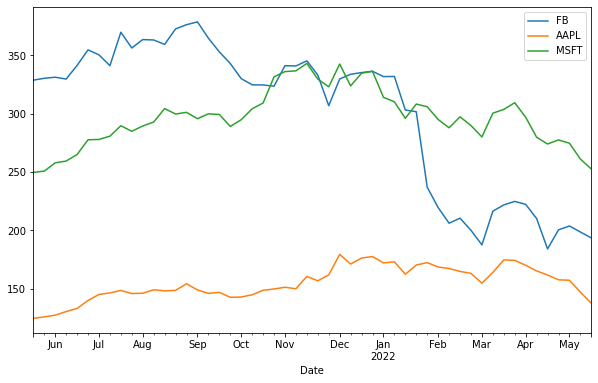We can use the other parameters provided by the `plot()` method to add more details to a plot, like this:

``df.plot(y='FB', figsize=(10,6), title='Facebook Stock', ylabel='USD')``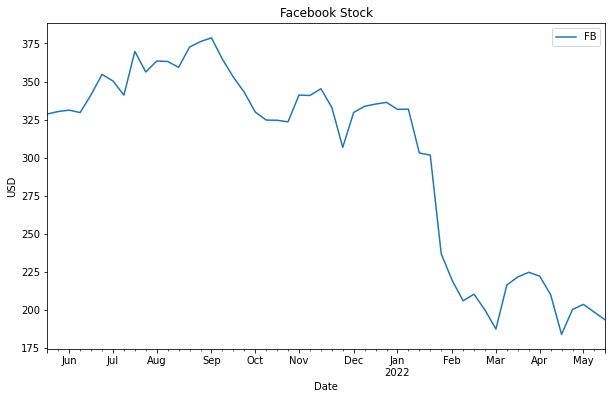As we see in the figure, the `title` argument adds a title to the plot, and the `ylabel` sets a label for the y-axis of the plot. The plot’s legend display by default, however, we may set the `legend` argument to `false` to hide the legend.

### Bar Plot

A bar chart is a basic visualization for comparing values between data groups and representing categorical data with rectangular bars. This plot may include the count of a specific category or any defined value, and the lengths of the bars correspond to the values they represent.

In the following example, we’ll create a bar chart based on the average monthly stock price to compare the average stock price of each company to others in a particular month. To do so, first, we need to resample data by month-end and then use the `mean()` method to calculate the average stock price in each month. We also select the last three months of data, like this:

``````df_3Months = df.resample(rule='M').mean()[-3:]
print(df_3Months)``````
``````                  MSFT          FB        AAPL
Date
2022-03-31  298.400002  212.692505  166.934998
2022-04-30  282.087494  204.272499  163.704994
2022-05-31  262.803335  198.643331  147.326665``````

Now, we’re ready to create a bar chart based on the aggregated data by assigning the `bar` string value to the `kind` argument:

``df_3Months.plot(kind='bar', figsize=(10,6), ylabel='Price')``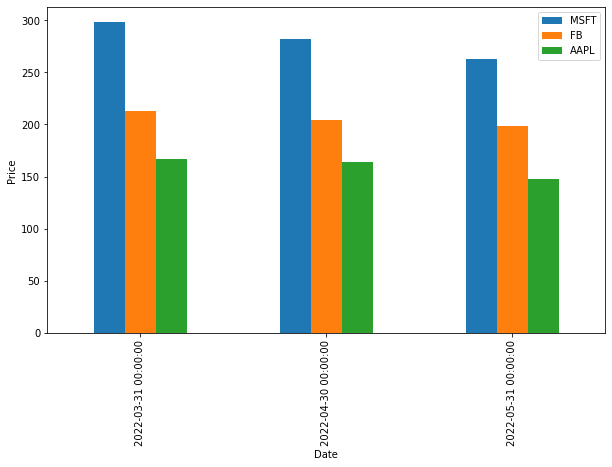We can create horizontal bar charts by assigning the `barh` string value to the `kind` argument. Let’s do it:

``df_3Months.plot(kind='barh', figsize=(9,6))``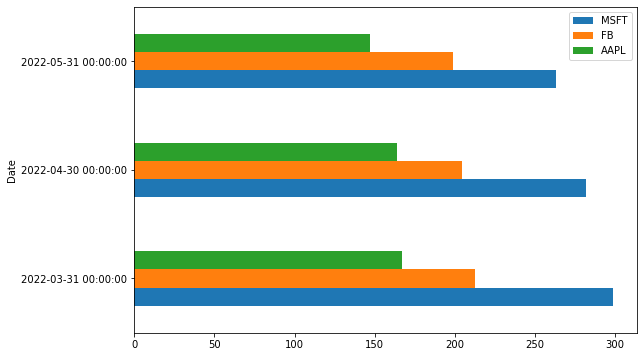We can also plot the data on the stacked vertical or horizontal bar charts, which represent different groups on top of each other. The height of the resulting bar shows the combined result of the groups. To create a stacked bar chart we need to assign `True` to the `stacked` argument, like this:

``df_3Months.plot(kind='bar', stacked=True, figsize=(9,6))``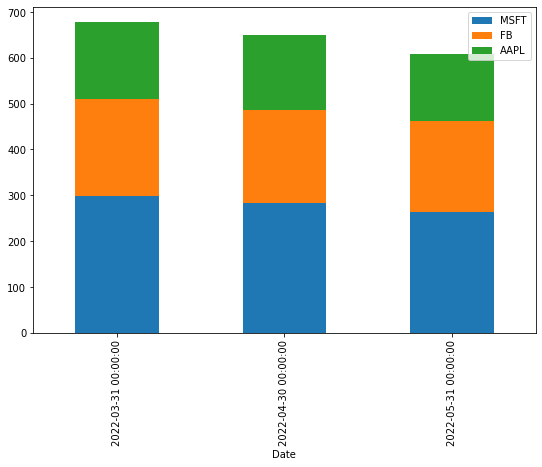### Histogram

A histogram is a type of bar chart that represents the distribution of numerical data where the x-axis represents the bin ranges while the y-axis represents the data frequency within a certain interval.

``df[['MSFT', 'FB']].plot(kind='hist', bins=25, alpha=0.6, figsize=(9,6))``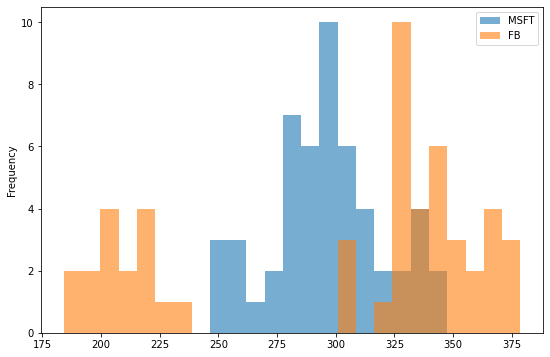In the example above, the `bins` argument specifies the number of bin intervals, and the `alpha` argument specifies the degree of transparency.

A histogram can also be stacked. Let’s try it out:

``df[['MSFT', 'FB']].plot(kind='hist', bins=25, alpha=0.6, stacked=True, figsize=(9,6))``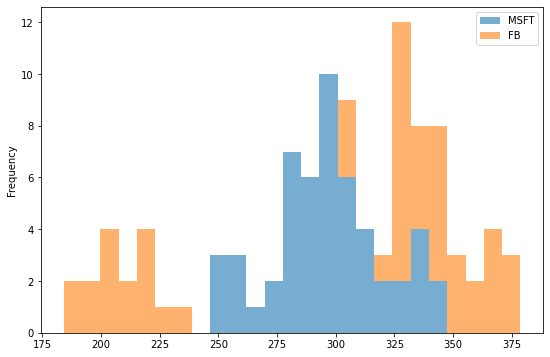### Box Plot

A box plot consists of three quartiles and two whiskers that summarize the data in a set of indicators: minimum, first quartile, median, third quartile, and maximum values. A box plot conveys useful information, such as the interquartile range (IQR), the median, and the outliers of each data group. Let’s see how it works:

``df.plot(kind='box', figsize=(9,6))``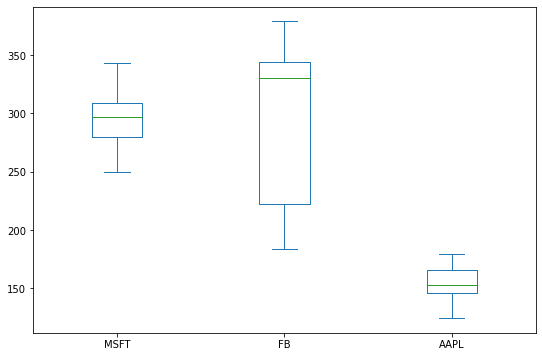We can create horizontal box plots, like horizontal bar charts, by assigning `False` to the `vert` argument. Like this:

``df.plot(kind='box', vert=False, figsize=(9,6))``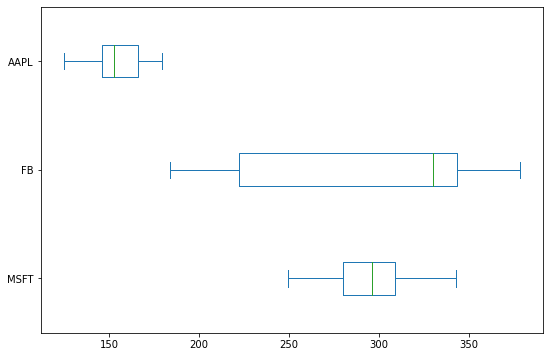### Area Plot

An area plot is an extension of a line chart that fills the region between the line chart and the x-axis with a color. If more than one area chart displays in the same plot, different colors distinguish different area charts. Let’s try it out:

``df.plot(kind='area', figsize=(9,6))``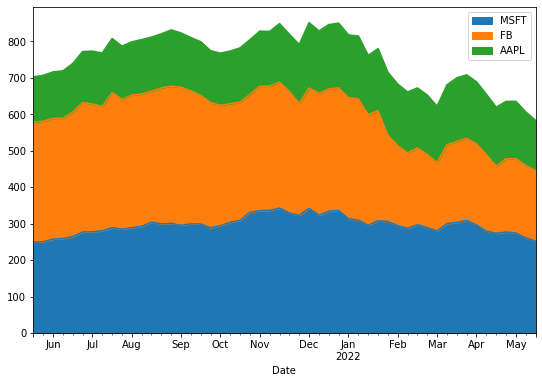The Pandas `plot()` method creates a stacked area plot by default. It’s a common task to unstack the area chart by assigning `False` to the `stacked` argument:

``df.plot(kind='area', stacked=False, figsize=(9,6))``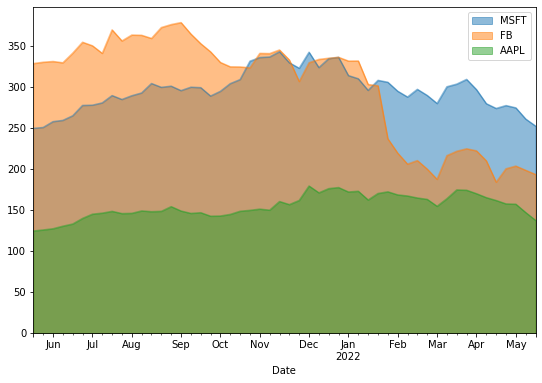### Pie Plot

If we’re interested in ratios, a pie plot is a great proportional representation of numerical data in a column. The following example shows the average Apple stock price distribution over the previous three months:

``````df_3Months.index=['March', 'April', 'May']
df_3Months.plot(kind='pie', y='AAPL', legend=False, autopct='%.f')``````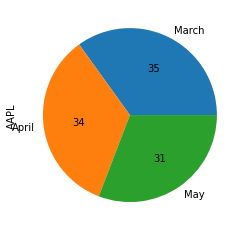A legend will display on pie plots by default, so we assigned `False` to the `legend` keyword to hide the legend.

The new keyword argument in the code above is `autopct`, which shows the percent value on the pie chart slices.

If we want to represent the data of all the columns in multiple pie charts as subplots, we can assign `True` to the `subplots` argument, like this:

``df_3Months.plot(kind='pie', legend=False, autopct='%.f', subplots=True, figsize=(14,8))``
``````array([, ,
], dtype=object)``````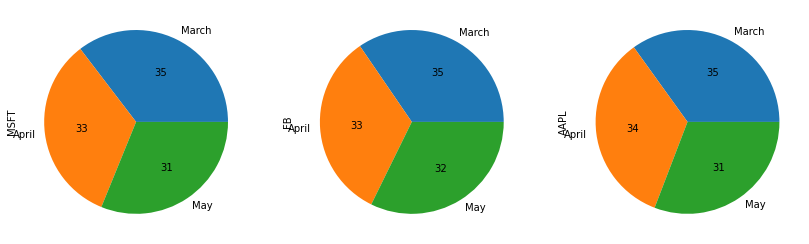### Scatter Plot

Scatter plots plot data points on the x and y axes to show the correlation between two variables. Like this:

``df.plot(kind='scatter', x='MSFT', y='AAPL', figsize=(9,6), color='Green')``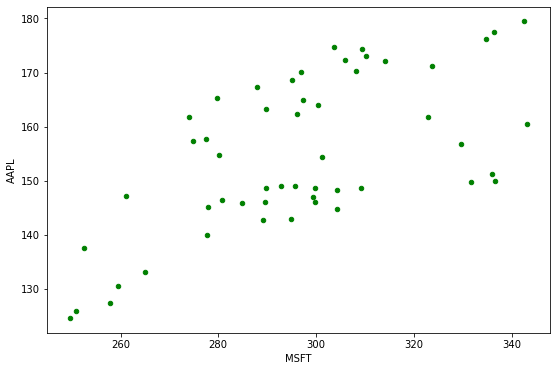As we see in the plot above, the scatter plot shows the relationship between Microsoft and Apple stock prices.

### Hexbin Plot

When the data is very dense, a hexagon bin plot, also known as a hexbin plot, can be an alternative to a scatter plot. In other words, when the number of data points is enormous, and each data point can’t be plotted separately, it’s better to use this kind of plot that represents data in the form of a honeycomb. Also, the color of each hexbin defines the density of data points in that range.

``df.plot(kind='hexbin', x='MSFT', y='AAPL', gridsize=10, figsize=(10,6))``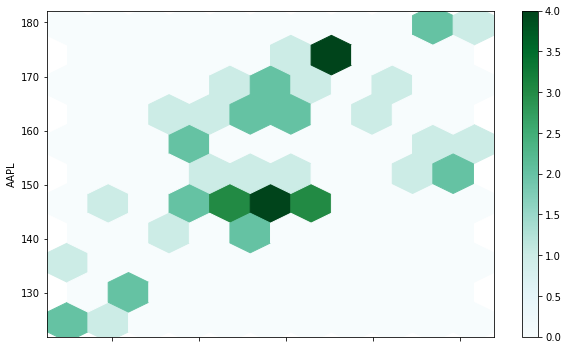The `gridsize` argument specifies the number of hexagons in the x-direction. A larger grid size means more and smaller bins. The default value of the `gridsize` argument is 100.

### KDE Plot

The last plot we want to discuss in this tutorial is the Kernel Density Estimate, also known as KDE, which visualizes the probability density of a continuous and non-parametric data variable. This plot uses Gaussian kernels to estimate the probability density function (PDF) internally. Let’s try it out:

``df.plot(kind='kde')``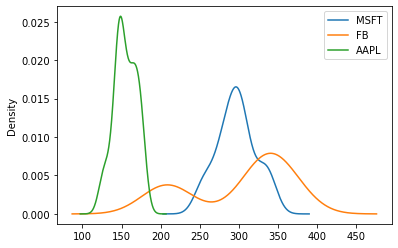We’re can also specify the bandwidth that affects the plot smoothness in the KDE plot, like this:

``df.plot(kind='kde', bw_method=0.1)``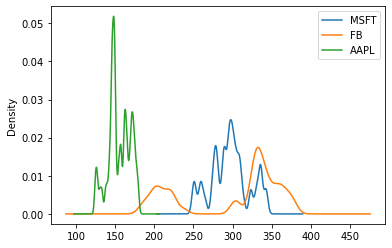``df.plot(kind='kde', bw_method=1)``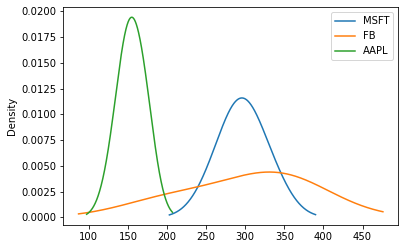As we can see, selecting a small bandwidth leads to under-smoothing, which means the density plot appears as a combination of individual peaks. On the contrary, a huge bandwidth leads to over-smoothing, which means the density plot appears as unimodal distribution.

## Summary

In this tutorial, we discussed the capabilities of the Pandas library as an easy-to-learn and straightforward data visualization tool. Then, we covered all the plots provided in Pandas by implementing some examples with very few lines of code.